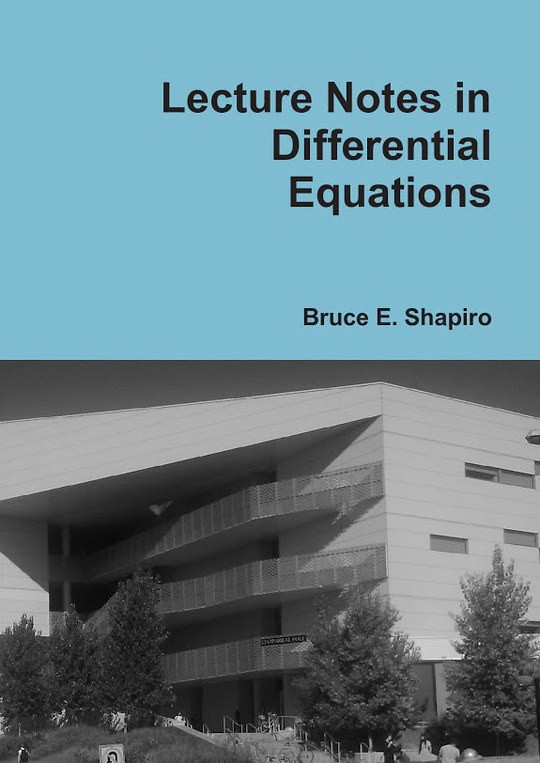Free

# Lecture Notes in Differential Equations

By Bruce Shapiro
Free
Book Description

These lecture notes on differential equations are based on the author's experience teaching Math 280 and Math 351 at California State University, Northridge since 2000. The content of Math 280 is more applied (solving equations) and Math 351 is more theoretical (existence and uniqueness) but the author has attempted to integrate the material together in the notes in a logical order and selects material from each section for each class.

The subject matter is classical differential equations and many of the exciting topics that could be covered in an introductory class, such as nonlinear systems analysis, bifurcations, chaos, delay equations, and difference equations are omitted in favor of providing a solid grounding the basics.

The book features some contributed drawings that are charming and humorous.

Printed copies available at Lulu.

• Front Cover
• Preface
• Basic Concepts
• A Geometric View
• Separable Equations
• Linear Equations
• Bernoulli Equations
• Exponential Relaxation
• Autonomous ODES
• Homogeneous Equations
• Exact Equations
• Integrating Factors
• Picard Iteration
• Existence of Solutions*
• Uniqueness of Solutions*
• Review of Linear Algebra
• Linear Operators and Vector Spaces
• Linear Eqs. w/ Const. Coefficents
• Some Special Substitutions
• Complex Roots
• Method of Undetermined Coefficients
• The Wronskian
• Reduction of Order
• Non-homog. Eqs.w/ Const. Coeff.
• Method of Annihilators
• Variation of Parameters
• Harmonic Oscillations
• General Existence Theory*
• Higher Order Equations
• Series Solutions
• Regular Singularities
• The Method of Frobenius
• Linear Systems
• The Laplace Transform
• Numerical Methods
• Critical Points
• Table of Integrals
• Table of Laplace Transforms
• Summary of Methods
• Bibliography
No review for this book yet, be the first to review.
No comment for this book yet, be the first to comment
Also Available On
Categories
Curated Lists
• #### Free Machine Learning Books

11 Books

Pattern Recognition and Machine Learning (Information Science and Statistics)
by Christopher M. Bishop
Data mining
by I. H. Witten
The Elements of Statistical Learning: Data Mining, Inference, and Prediction
by Various
See more...
• #### Free Chemistry Textbooks

9 Books

CK-12 Chemistry
by Various
Concept Development Studies in Chemistry
by John Hutchinson
An Introduction to Chemistry - Atoms First
by Mark Bishop
See more...
• #### Free Mathematics Textbooks

21 Books

Microsoft Word - How to Use Advanced Algebra II.doc
by Jonathan Emmons
Advanced Algebra II: Activities and Homework
by Kenny Felder
de2de
by
See more...
• #### Free Children Books

38 Books

The Sun Who Lost His Way
by
Tania is a Detective
by Kanika G
Firenze_s-Light
by
See more...
• #### Free Java Books

10 Books

Java 3D Programming
by Daniel Selman
The Java EE 6 Tutorial
by Oracle Corporation
JavaKid811
by
See more...